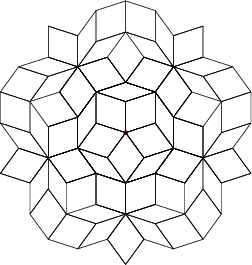# Laurentiu PaunescuProfessor in the School of Mathematics and Statistics at the University of Sydney.

Postal address: Prof Laurentiu Paunescu School of Mathematics and Statistics F07 University of Sydney NSW 2006 Australia Room 721 Carslaw Building laurent@maths.usyd.edu.au +61 2 9351 2969 +61 2 9351 4534

## Teaching

### Workshop Organiser

Australian-Japanese Real and Complex Singularities Workshop RCSW

#### Research Interests

Domains of Research:
• Real and Complex Singularities, Stratifications.
• Real and Complex Algebraic Geometry.
My main research interests are in real and complex singularities,in stratification theory and in real and complex polynomials.

I am interested in geometric criteria allowing us to tell when we can ignore higher order terms in a weighted (or Newton) Taylor expansion of an analytic map, without changing the topological type defined by it. These can be used to understand the lists of V.I. Arnold and C.T.C. Wall of simple and unimodular germs.

Another problem concerns the classification of real singularities via "blow-analytic" equivalence.

I found examples of pathological blow-analytic homeomorphisms, which shows that we have to strenghten the definition of this kind of homeomorphisms in order that the classification obtained by using them to be an interesting one. To develop "Calculus" for blow-analytic maps is an exciting and difficult area of research.

Another direction in my research concerns the interplay between the real Milnor fibre and the complex Milnor fibre. I established a relation between the Milnor fibre of a holomorphic function h=f+ig, h : Cn -> C, and the Milnor fibre of its real part f, f : R2n -> R. In particular, when n = 3, we get easy equations for a connected sum of k copies of S2 x S2, just taking the real part of Ak singularities h = x2 + y2 + zk+1.

In a paper with A. Dimca, we investigate the action of the dihedral group on the cohomology of the Milnor fibre associated to the complexification of a real polynomial. The main idea of this paper is used and extended by McCrory and Parusinski in a recent paper.

In a paper with S. Papadima, we study the negative derivations of the quasihomogeneous complete intersections C[x1,...,xn] / (f1,...,fn), and we prove a conjecture, due to Halperin, in the generic case when C[x1,...,xn] / (f1,...,fn-1) is reduced (here we assume deg f1 <= deg f2 <= ... <= deg fn).

In a paper with A. Dimca and J. Hillman, we study the topology of the real projective hypersurfaces using complex singularities. In particular we give very simple polynomial equations for some classic surfaces embedded in the real projective space RP3.

Recently with A. Zaharia, we produced very interesting examples of complex polynomials in n > 2 variables, which are components of algebraic automorphisms of Cn, but with bad behaviour at infinity. This is in deep contradiction with the case n = 2, as for example can be seen in the work of Lê.

In another paper with A. Dimca, we give an important result concerning the connectivity of the affine complex hypersurfaces, looking only at the top two succesive terms in a weighted Taylor expansion of a given polynomial.

I am a member of the Geometry and Topology group.

Publications